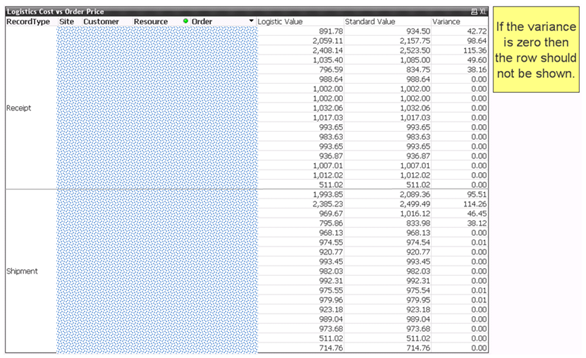# QlikView Layout & Visualizations

Discussion Board for collaboration on QlikView Layout & Visualizations.

Announcements
Leverage your QlikView investment to modernize BI – see how! Join Group
cancel
Showing results for
Did you mean:
HighlightedNot applicable

## Expression Filtration Issue

Goal - The chart is to only display rows where expression value is not equal to x.Suppress Zero - I wrapped all the equations within expression boxes in the chart to say, if x = 0 then display 0, or else do existing equation. This worked but it is not healthy looking. It is a bit of a hack so I dropped it!

Code Behind - I know I can achieve the goal if I do the equation in the code behind and quite tidily too but I wish it could be easier than this, for example, a click of a button or writing a code into a separate expression field in the chart with a command to not display the line.

Is there away around it other than those stated above?

Is this a limit of Qlikview I am encountering?

Thanks,

Andrew.

Tags (2)
2 Replies
HighlightedPartner

## Expression Filtration Issue

Hi Andrew,

You can use the expression1 Logistic Value= If(Sum(Logistic Value)<>Sum(Standard Value),Sum(Logistic Value)

Expression2:  If(Sum(Logistic Value)<>Sum(Standard Value),Sum(Standard Value)

Expressio3 = Column1-column2

HighlightedMVP & Luminary

## Expression Filtration Issue

Hi,

Use the following expression

Logistic Value =If([VarianceExpressionName] = 0, 0, LogisticValueExpression) //If variance is 0 then 0

Standard Value = If([VarianceExpressionName] = 0, 0, StandardValueExpression) //If variance is 0 then 0

VarianceExpression = VarianceExpression

If all the expressions in straight/pivot table returns 0 then the row would be automatically removed by qlikview.

Regards,

Jagan.